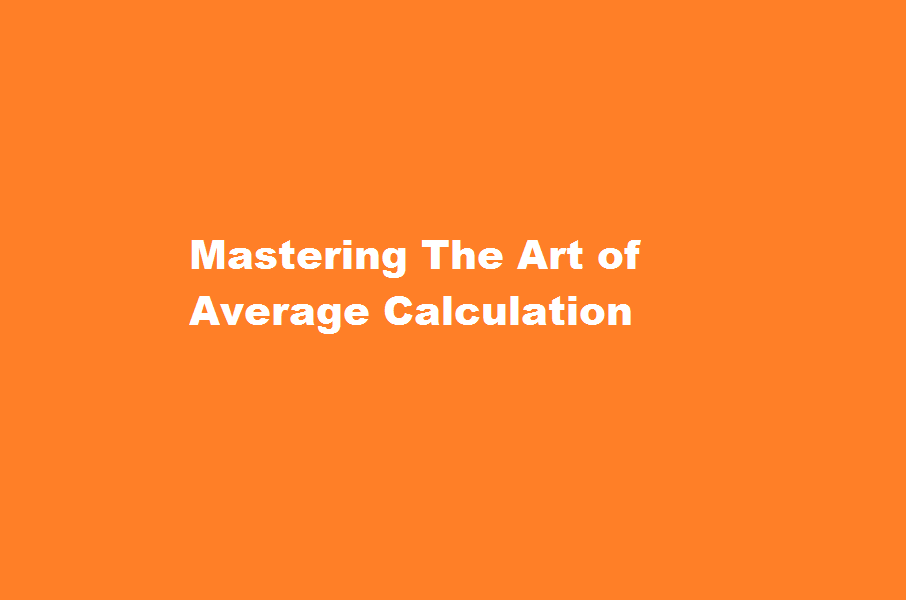December 4, 2023
[stock-market-ticker]

# Mastering The Art of Average CalculationContents

## Introduction

Calculating averages is a fundamental mathematical concept that finds applications in various fields. Whether you’re analysing data, assessing performance, or simply trying to understand a situation better, understanding how to calculate averages is essential. In this article, we will explore the methods and techniques for calculating averages and provide practical examples along the way.

## Understanding the Average

The average, also known as the arithmetic mean, represents the central tendency of a set of values. It is calculated by summing all the values and dividing the sum by the number of values. Averages are commonly used to summarise data and provide insights into trends and patterns. However, it’s crucial to consider the context and limitations of the data being averaged to draw accurate conclusions.

Also Read  Effective Liquidity Management Strategies Mitigating Liquidity Risks in Banks

## Calculating the Mean

To calculate the mean of a set of numbers, follow these steps

• Add up all the numbers in the set.
• Count the total number of values.
• Divide the sum by the count to find the mean.

For example, let’s calculate the mean of the following dataset 5, 7, 10, 12, and 15.

• Sum: 5 + 7 + 10 + 12 + 15 = 49
• Count: There are five numbers in the set.
• Mean: 49 ÷ 5 = 9.8

The mean, in this case, is 9.8. It represents the average value of the dataset.

## Weighted Average

In some scenarios, assigning weights to different values can be more appropriate. This approach is known as a weighted average. Each value is multiplied by its respective weight before summing and dividing by the total weight.

Also Read  Best Practices To Mitigate Web3 Security Risks

For instance, consider a situation where grades in a course are assigned different weights based on their importance. Let’s say the weights assigned to the grades are 0.3, 0.4, and 0.3, respectively, for quizzes, assignments, and the final exam. The scores obtained in each category are 85, 90, and 80, respectively.

## To calculate the weighted average

• Multiply each score by its corresponding weight: 85 × 0.3, 90 × 0.4, 80 × 0.3.
• Sum the weighted scores: (85 × 0.3) + (90 × 0.4) + (80 × 0.3) = 25.5 + 36 + 24 = 85.5.
• Sum the weights: 0.3 + 0.4 + 0.3 = 1.
• Divide the sum of the weighted scores by the sum of the weights: 85.5 ÷ 1 = 85.5.
• The weighted average, in this case, is 85.5.

### What are the 3 ways to calculate average?

There are three main types of average: mean, median and mode. Each of these techniques works slightly differently and often results in slightly different typical values.

Also Read  A Guide to Securing a Job in The Central Bank of India

### What are the 5 types of average?

arithmetic mean, weighted mean, geometric mean (GM) and harmonic mean (HM).

## Conclusion

Calculating averages is an essential skill in various aspects of life, from academics to business. Whether you’re analysing numerical data, assessing performance, or estimating trends, the average provides a valuable summary. Remember, the mean represents the typical value in a dataset, while the weighted average accounts for the importance of different values. By following the appropriate method, you can derive accurate and meaningful insights. So, the next time you encounter a set of numbers, put your knowledge of average calculation into practice and unlock the power of central tendency analysis.